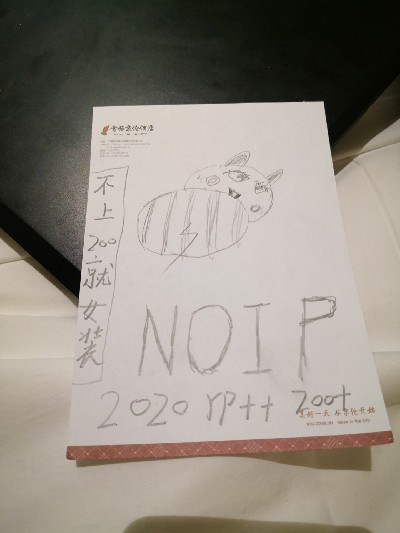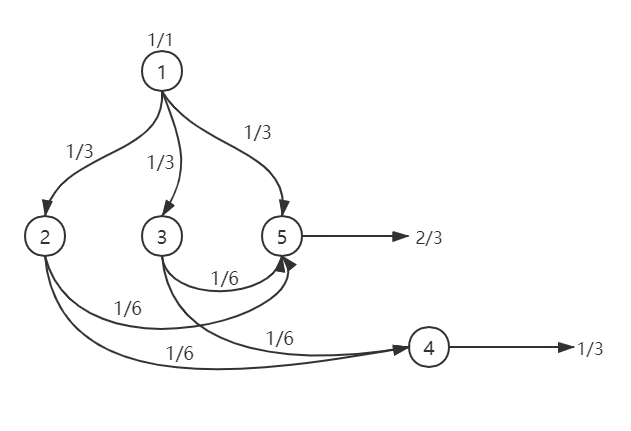# NOIP 2020 游记 & 题解

## 游记$\text{y\color{red}{ouwike}}$ 在考前立的 $flag$▲ 选手胸牌长这样▲ 早上还照了一张合照

## 题解：排水系统（water）

### 输入输出样例

5 1
3 2 3 5
2 4 5
2 5 4
0
0

1 3
2 3

$1$ 号结点是接收口，$4, 5$ 号结点没有排出管道，因此是最终排水口。
$1$ 吨污水流入 $1$ 号结点后，均等地流向 $2, 3, 5$ 号结点，三个结点各流入 $\frac{1}{3}$ 吨污水。
$2$ 号结点流入的 $\frac{1}{3}$ 吨污水将均等地流向 $4, 5$ 号结点，两结点各流入 $\frac{1}{6}$ 吨污水。
$3$ 号结点流入的 $\frac{1}{3}$ 吨污水将均等地流向 $4, 5$ 号结点，两结点各流入 $\frac{1}{6}$ 吨污水。

### 数据范围

$1 \sim 3$$10$$1$
$4 \sim 6$${10}^3$$1$
$7 \sim 8$${10}^5$$1$
$9 \sim 10$${10}^5$$10$

### 思路

$1 \sim m$ 节点开始向下搜索，如果搜到没有排水口的节点就更新节点的 $ans$P7113 Sample1

▲ 样例1的搜索过程

\begin{aligned} &\frac{2}{3} \div 4 \\ =& \frac{2}{3} \times \frac{1}{4} \\ =& \frac{2}{3 \times 4}\\ =& \frac{2}{12} =& \frac{1}{6} \end{aligned}

\begin{aligned} &\frac{2}{3} + \frac{3}{4} \\ =& \frac{2 \times 4}{3 \times 4} + \frac{3\times 3}{3 \times 4}\\ =& \frac{2 \times 4 + 3 \times 4}{3\times 4}\\ =& \frac{20}{12}\\ =& \frac{5}{3} \end{aligned}

### 代码

#include<bits/stdc++.h>

using namespace std;

int n, m, d, t;
vector<int> u;
pair<long long, long long> ans;

long long gcd(long long a, long long b) {
if(b == 0) return a;
return gcd(b, a%b);
}

void dfs(int x, long long first, long long second) {
if(u[x].empty()) {
if(ans[x].first && ans[x].second) {
if(ans[x].second == second) {
ans[x].first += first;
long long tmp = gcd(ans[x].first, ans[x].second);
ans[x].first /= tmp;
ans[x].second /= tmp;
}
else {
first *= ans[x].second;
ans[x].first *= second;
ans[x].first += first;
ans[x].second *= second;
long long tmp = gcd(ans[x].first, ans[x].second);
ans[x].first /= tmp;
ans[x].second /= tmp;
}
}
else {
ans[x].first = first;
ans[x].second = second;
}
return;
}
int s = u[x].size();
second *= s;
for(int i = 0 ; i < s; i++) {
dfs(u[x][i], first, second);
}
}

int main() {
cin >> n >> m;
for(int i = 1 ; i <= n ; i++) {
cin >> d;
for(int j = 1 ; j <= d ; j++) {
cin >> t;
u[i].push_back(t);
}
}
for(int i = 1 ; i <= m ; i++) {
dfs(i, 1, 1);
}
for(int i = 1 ; i <= n ; i++) {
if(u[i].empty()) {
cout << ans[i].first << ' ' << ans[i].second << endl;
}
}
return 0;
}

AC代码

#include <bits/stdc++.h>

using namespace std;

int n, m, d, t;
vector<int> u;
pair<__int128, __int128> ans;

ostream& operator<<(ostream &__ostream, __int128 __n) {
string __o;
while (__n) {
__o.push_back(__n%10+'0');
__n /= 10;
}
reverse(__o.begin(), __o.end());
return __ostream << __o;
}

__int128 gcd(__int128 a, __int128 b) {
if (b == 0) return a;
return gcd(b, a % b);
}

void dfs(int x, __int128 first, __int128 second) {
if (u[x].empty()) {
if (ans[x].first && ans[x].second) {
if (ans[x].second == second) {
ans[x].first += first;
__int128 tmp = gcd(ans[x].first, ans[x].second);
ans[x].first /= tmp;
ans[x].second /= tmp;
}
else {
first *= ans[x].second;
ans[x].first *= second;
ans[x].first += first;
ans[x].second *= second;
__int128 tmp = gcd(ans[x].first, ans[x].second);
ans[x].first /= tmp;
ans[x].second /= tmp;
}
}
else {
ans[x].first = first;
ans[x].second = second;
}
return;
}
int s = u[x].size();
second *= s;
for (int i = 0; i < s; i++) {
dfs(u[x][i], first, second);
}
}

int main() {
cin >> n >> m;
for (int i = 1; i <= n; i++) {
cin >> d;
for (int j = 1; j <= d; j++) {
cin >> t;
u[i].push_back(t);
}
}
for (int i = 1; i <= m; i++) {
dfs(i, 1, 1);
}
for (int i = 1; i <= n; i++) {
if (u[i].empty()) {
cout << ans[i].first << ' ' << ans[i].second << endl;
}
}
return 0;
}
NOIP 2020 游记 & 题解

2020-12-05